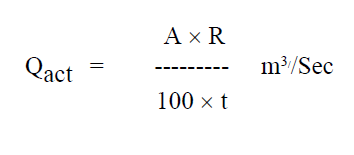# Orifice calibration

The orifice meter is a simple device that measures the flow in a closed stream. The orifice meter also works on the same principle as the venturi meter, that is, by reducing the cross-sectional area of the flow path, a pressure difference develops between the two sections before and after the hole is developed and the Measurement of the pressure difference allows the determination of discharge through the pipeline.

The orifice plates are widely used in flow installation as it is cheaper and utilizes only less place.

## Calibration of Orifice meter:

### Setup:

Orifice meter fitted across a pipeline leading to a collecting tank, Stop Watch, U-Tube manometer connected across entry and throat sections etc.

• First measure the diameter of the inlet of pipe (d(1)) and the diameter of orifice d(0).

The measurement of pressure difference is used to find the discharge.

Theoretical discharge:

Where, a1 = Area of Intel section a2 = Area of vena-contracta Loss of head ‘H’ = 12.6 x h in m

Where, h = Manometer differential head in mm of Hg.

Actual discharge:Where,

A = Area of measuring tank in m2 R = Rise of water level for time‘t’ secs in m. t = time taken in seconds for ‘R’ mm rise of water

The coefficient of discharge of the Orificemeter, Cd = Qa/Qth

The graph of equation (1) can made linear by taking logarithms on both side.

ie, log Q = log K + n log H

## Procedure for orifice calculation:

• Make sure that the apparatus are free from Dust.

• Note the diameter of inlet of pipe (d(1)) and the diameter of orifice d(0).

• Note the density of the manometer liquid that is mercury and that of the liquid that flows through the pipe that is water.

• Connect the U-tube pressure gauge to the pressure gauges of the orifice meter, one end in the inlet section and the other end in the section where the water jet leaves the orifice forming a vein contracted.

• Start the flow and adjust the control valve in the pipeline to obtain the required discharge.

• Measure the pressure difference (Hm) between two sections of the orifice meter using a mercury gauge in a U-tube.

• Measure the flow velocity, that is, the actual discharge (Qact) through the hole in the orifice by collecting the water in the collection tank for a specific period of time.

• Change the flow rate by adjusting the control valve and repeat the process at least five times.

## Table for calculation:

OPENINGS MANOMETER
mm of Hg
TIME
TAKEN
FOR
20cm
RISE OF
WATER t
'sec
AREA
(A)
AREA
(a1)
AREA
(a2)
Qth Qact Cd
D= 25mm
d=12.5mm